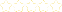• > Lora Krantz
• > Solving Equations
+# Solving Equations

Owner: Lora Krantz
##### Description:
Learn how to solve single step algebraic equations.

### Your Quiz Points in this Playlist

Total Possible
0
4 Tutorials in this Playlist

Author: Luis
Rating:(36)
This tutorial has a quiz
•• -

#### Solving Subtraction Equations

Author: Ms. Hess
Rating:(34)
This tutorial has a quiz
•• -

#### Solving Multiplication Equations

Author: Dr. C
Rating:(28)
This tutorial has a quiz
•• -

#### Solving Division Equations

Author: JoshHansen
Rating:(30)
This tutorial has a quiz
•• -